# 浮点数运算丢失精度

print(sys.float_info.max - 1.0)
print(sys.float_info.max)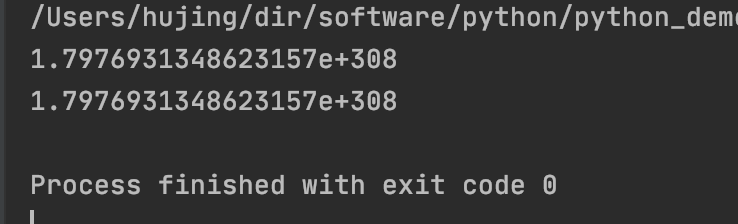a = sys.float_info.max - 1.0
b = sys.float_info.max
print(a == b)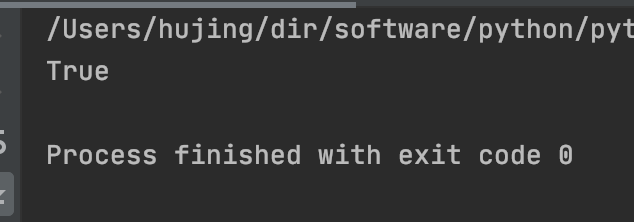# 小数的存储

### 2. 浮点数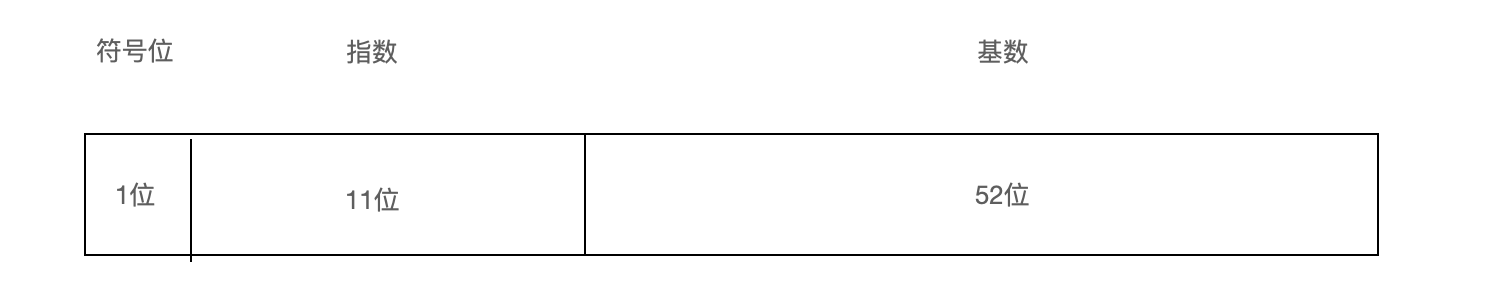• 符号位: 标识数字的正负
• 指数: 2^n. 其中这个指数是分正负的哦, 也可以理解为小数点偏移量.
• 基数: 规定基数是一个大于等于1, 小于2的数字, 也就是基数前面有一个隐含的默认1, 基数标识小数点后面的内容

• 当指数为全0的时候, 若基数为全0, 则表示0.
• 当指数为全1的时候, 表示无穷大.

# 再看

1.0*10^4 + 1.0*10^2 要转换成: 1.0*10^4 + 0.01*10^4

a = 1.0
b = 0.12345678
c = 0.11111111

s = 0.0
s += a
s += b
s += c

print(s)

s +=  10000000.0
s += -10000000.0
print(s)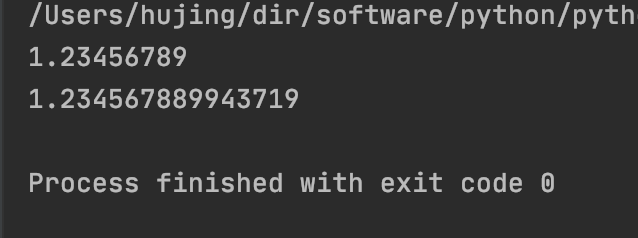a = 1.0
b = 0.12345678
c = 0.11111111

s = 0.0
s += a
s +=  10000000.0
s += -10000000.0
s += b
s += c

print(s)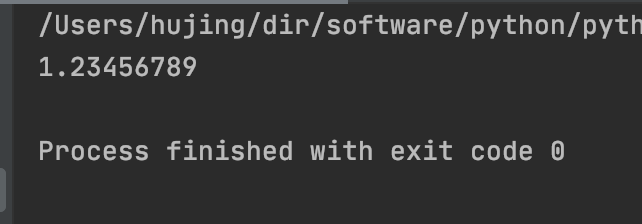0 评论

0